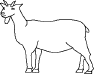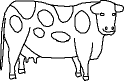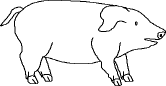EnchantedLearning.com is a user-supported site.
As a bonus, site members have access to a banner-ad-free version of the site, with print-friendly pages.

 You might also like: Divide Groups of Bugs Worksheet Printout Simple Math: Farm and Farm Animals Divide Fish by Five Worksheet Printout Divide Eight Birds Worksheet Printout Divide Ten Pets Worksheet Printout Today's featured page: Apple Quiz: Student Research Activity

 Go to the AnswersMath Pages EnchantedLearning.com Divide Twelve Farm Animals Visual Division PagesDivision Pages

 Divide the goats into two equal groups.How many goats are there? How many groups of goats are there? How many goats are in each group?
16 ÷ 2 =

 Divide the cows into four equal groups.How many cows are there? How many groups of cows are there? How many cows are in each group?
16 ÷ 4 =

 Divide the pigs into eight equal groups.How many pigs are there? How many groups of pigs are there? How many pigs are in each group?
16 ÷ 8 =

## Enchanted Learning Search

 Search the Enchanted Learning website for: